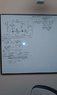# Circuit with 2 batteries and 6 resistors

riane

## Homework Statement

A circuit is constructed with six resistors and two batteries as shown. The battery voltages are V1 = 18 V and V2 = 12 V. The positive terminals are indicated with a + sign, The values for the resistors are: R1 = R5 = 73 Ω, R2 = R6 = 121 Ω R3 = 62 Ω, and R4 = 92 Ω. The positive directions for the currents I1, I2 and I3 are indicated by the directions of the arrows. What is V4, I1, I2, and I3?

V=IR

## The Attempt at a Solution

-I3R3+I1R1-ΔV1=0
+I6R6-ΔV2+I2R2+I1R1=0
-I1R1-I2R2-I5R5-I4R4-I6R6=0
-ΔV1-I3R3-I2R2+ΔV2-I6R6=0
-ΔV2-I5R5-I4R4=0
-I4R4-I6R6-ΔV1-I3R3-I2R2-I5R5=0

https://www.physicsforums.com/attachment.php?attachmentid=43882&d=1329194903

Mentor

## Homework Statement

A circuit is constructed with six resistors and two batteries as shown. The battery voltages are V1 = 18 V and V2 = 12 V. The positive terminals are indicated with a + sign, The values for the resistors are: R1 = R5 = 73 Ω, R2 = R6 = 121 Ω R3 = 62 Ω, and R4 = 92 Ω. The positive directions for the currents I1, I2 and I3 are indicated by the directions of the arrows. What is V4, I1, I2, and I3?

V=IR

## The Attempt at a Solution

-I3R3+I1R1-ΔV1=0
+I6R6-ΔV2+I2R2+I1R1=0
-I1R1-I2R2-I5R5-I4R4-I6R6=0
-ΔV1-I3R3-I2R2+ΔV2-I6R6=0
-ΔV2-I5R5-I4R4=0
-I4R4-I6R6-ΔV1-I3R3-I2R2-I5R5=0

https://www.physicsforums.com/attachment.php?attachmentid=43882&d=1329194903

You've written a number of KVL equations which appear to be correct assuming that the directions of the currents not specified in the diagram are chosen appropriately.

You should now consider writing some KCL equations at junctions so that you can eliminate superfluous currents (for example, note that I4 and I5 are really the same current since the branch they're in is series-connected. How are I2 and I6 related?

Once you've established the relationships between the currents you should be in a position to choose a subset of your equations and solve for the unknowns (simultaneous equations). Choose a set of equations such that each component appears at least once in the set.

Note: The placement of voltage source V2 effectively isolates the rightmost loop from the rest of the circuit. Can you see why?

riane
I already established the relationships between the currents: I2=I6 and I4=I5; I2=I1+I3; R2=R6

I already have worked out the problem using:
-I3R3+I1R1-ΔV1=0,
+I6R6-ΔV2+I2R2+I1R1=0, and I2=I1+I3, and I keep getting I1=.147 or .10 or .11, and all three answers for I1 are wrong. I see that V2 isolates the rightmost loop from the rest of the circuit, but I don't know what That is supposed to do for me. Is it supposed to give me I4 and I5 with the equation -ΔV2-I5R5-I4R4=0? If I have that, then how is it going to help me find I1 I2 and I3 any different than solving for individual variables like I already have above? I feel like I'm just going in circles.

Mentor
I already established the relationships between the currents: I2=I6 and I4=I5; I2=I1+I3; R2=R6

I already have worked out the problem using:
-I3R3+I1R1-ΔV1=0,
+I6R6-ΔV2+I2R2+I1R1=0, and I2=I1+I3, and I keep getting I1=.147 or .10 or .11, and all three answers for I1 are wrong. I see that V2 isolates the rightmost loop from the rest of the circuit, but I don't know what That is supposed to do for me. Is it supposed to give me I4 and I5 with the equation -ΔV2-I5R5-I4R4=0? If I have that, then how is it going to help me find I1 I2 and I3 any different than solving for individual variables like I already have above? I feel like I'm just going in circles.

The effect of V2 means that you can completely ignore R4 and R5 and their current when you are looking to find I1, I2, I3.

Can you show the steps you used to solve your equations to find I1? Maybe we can locate where things are going astray.

riane
Here is an attatched image of the work done in altering the equations and plugging in the unknowns.

#### Attachments

•homework image.jpg
15.3 KB · Views: 1,663
riane
Thank you!